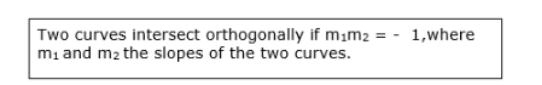Deepak Scored 45->99%ile with Bounce Back Crack Course. You can do it too!

# Show that the following set of curves intersect orthogonally:

Question:

Show that the following set of curves intersect orthogonally:

$x^{3}-3 x y^{2}=-2$ and $3 x^{2} y-y^{3}=2$

Solution:

Given:

Curves $x^{3}-3 x y^{2}=-2 \ldots$ (1)

$\& 3 x^{2} y-y^{3}=2 \ldots(2)$

Adding (1) \& (2), we get

$\Rightarrow x^{3}-3 x y^{2}+3 x^{2} y-y^{3}=-2+2$

$\Rightarrow x^{3}-3 x y^{2}+3 x^{2} y-y^{3}=-0$

$\Rightarrow(x-y)^{3}=0$

$\Rightarrow(x-y)=0$

$\Rightarrow x=y$

Substituting $x=y$ on $x^{3}-3 x y^{2}=-2$

$\Rightarrow x^{3}-3 x x \times x^{2}=-2$

$\Rightarrow x^{3}-3 x^{3}=-2$

$\Rightarrow-2 x^{3}=-2$

$\Rightarrow x^{3}=1$

$\Rightarrow x=1$

Since $x=y$

$y=1$

The point of intersection of two curves is $(1,1)$

First curve $x^{3}-3 x y^{2}=-2$

Differentiating above w.r.t $x$,

$\Rightarrow 3 x^{2}-3\left(1 \times y^{2}+x \times 2 y \frac{d y}{d x}\right)=0$

$\Rightarrow 3 x^{2}-3 y^{2}-6 x y \frac{d y}{d x}=0$

$\Rightarrow 3 x^{2}-3 y^{2}=6 x y \frac{d y}{d x}$

$\Rightarrow \frac{d y}{d x}=\frac{3 x^{2}-3 y^{2}}{6 x y}$

$\Rightarrow \frac{d y}{d x}=\frac{3\left(x^{2}-y^{2}\right)}{6 x y}$

$\Rightarrow m_{1}=\frac{\left(x^{2}-y^{2}\right)}{2 x y}$       .....(3)

Second curve $3 x^{2} y-y^{3}=2$

Differentiating above w.r.t $x$,

$\Rightarrow 3\left(2 x \times y+x^{2} \times \frac{d y}{d x}\right)-3 y^{2} \frac{d y}{d x}=0$

$\Rightarrow 6 x y+3 x^{2} \frac{d y}{d x}-3 y^{2} \frac{d y}{d x}=0$

$\Rightarrow 6 x y+\left(3 x^{2}-3 y^{2}\right) \frac{d y}{d x}=0$

$\Rightarrow \frac{d y}{d x}=\frac{-6 x y}{3 x^{2}-3 y^{2}}$

$\Rightarrow \frac{d y}{d x}=\frac{-2 x y}{x^{2}-y^{2}}$

$\Rightarrow m_{2}=\frac{-2 x y}{x^{2}-y^{2}} \ldots(4)$

When $m_{1}=\frac{\left(x^{2}-y^{2}\right)}{2 x y} \& m_{2}=\frac{-2 x y}{x^{2}-y^{2}}$$\Rightarrow \frac{\left(x^{2}-y^{2}\right)}{2 x y} \times \frac{-2 x y}{x^{2}-y^{2}}=-1$

$\therefore$ Two curves $x^{3}-3 x y^{2}=-2 \& 3 x^{2} y-y^{3}=2$ intersect orthogonally.